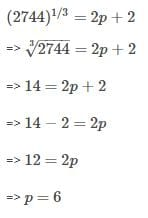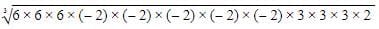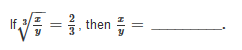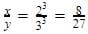UPSC  >  Olympiad Test: Cubes And Cube Roots

# Olympiad Test: Cubes And Cube Roots - UPSC

Test Description

## 20 Questions MCQ Test CSAT Preparation - Olympiad Test: Cubes And Cube Roots

Olympiad Test: Cubes And Cube Roots for UPSC 2023 is part of CSAT Preparation preparation. The Olympiad Test: Cubes And Cube Roots questions and answers have been prepared according to the UPSC exam syllabus.The Olympiad Test: Cubes And Cube Roots MCQs are made for UPSC 2023 Exam. Find important definitions, questions, notes, meanings, examples, exercises, MCQs and online tests for Olympiad Test: Cubes And Cube Roots below.
Solutions of Olympiad Test: Cubes And Cube Roots questions in English are available as part of our CSAT Preparation for UPSC & Olympiad Test: Cubes And Cube Roots solutions in Hindi for CSAT Preparation course. Download more important topics, notes, lectures and mock test series for UPSC Exam by signing up for free. Attempt Olympiad Test: Cubes And Cube Roots | 20 questions in 20 minutes | Mock test for UPSC preparation | Free important questions MCQ to study CSAT Preparation for UPSC Exam | Download free PDF with solutions
 1 Crore+ students have signed up on EduRev. Have you?
Olympiad Test: Cubes And Cube Roots - Question 1

### A natural number is said to be a perfect cube, if it is the cube of some _________.

Detailed Solution for Olympiad Test: Cubes And Cube Roots - Question 1

The cube of a natural number is always a natural number.

Olympiad Test: Cubes And Cube Roots - Question 2

### The cube of 23 is ___________

Detailed Solution for Olympiad Test: Cubes And Cube Roots - Question 2

23= 23×23×23 = 12167

Olympiad Test: Cubes And Cube Roots - Question 3

### Find the cube of 75.

Olympiad Test: Cubes And Cube Roots - Question 4

If n = m × m × m = m3, where m is an integer, then n is a perfect cube and the number m is called the __________ of n.

Olympiad Test: Cubes And Cube Roots - Question 5

If (2744)1/3 = 2p+2, then the value of P is

Detailed Solution for Olympiad Test: Cubes And Cube Roots - Question 5Olympiad Test: Cubes And Cube Roots - Question 6

The cube root of 13824 is __________.

Detailed Solution for Olympiad Test: Cubes And Cube Roots - Question 6
By prime factorisation:
13824 is (2x2x2)x(2x2x2)x(2x2x2)x(3x3x3)
Making pairs of three three numbers
which gives 2x2x2x3=24
Therefore cube root of 13824 is 24
Olympiad Test: Cubes And Cube Roots - Question 7

The square of a natural number subtracts from its cube comes 100. The number is __________.

Olympiad Test: Cubes And Cube Roots - Question 8

Find the cube root of 0.001331.

Olympiad Test: Cubes And Cube Roots - Question 9

Find the smallest number by which 54 must be multiplied so that the product is a perfect cube.

Olympiad Test: Cubes And Cube Roots - Question 10

Find the ones digit of cube root of 2197.

Detailed Solution for Olympiad Test: Cubes And Cube Roots - Question 10

2197

Unit's place of 2197 = 7

The ones digit of cube root of 2197 will be 3 as 3= 27 which has 7 as unit's place

Olympiad Test: Cubes And Cube Roots - Question 11

Find the cube root of -5832.

Olympiad Test: Cubes And Cube Roots - Question 12

The cube root of the 216 x (−32) x 54 is ________

Detailed Solution for Olympiad Test: Cubes And Cube Roots - Question 12

We have 216 x (-32) x 54.
Applying prime factorisation giveswhich gives  -(6 x 2 x 2 x 3)= -72.

Olympiad Test: Cubes And Cube Roots - Question 13

If x is ones digit and y is tens digit of a two digit number, then the cube of the number will be _________.

Olympiad Test: Cubes And Cube Roots - Question 14

If 72×K is a perfect cube, find the value of K.

Detailed Solution for Olympiad Test: Cubes And Cube Roots - Question 14

72 = 2×2×2×3×3

If  K = 3, 72×K = 72 × 3 = 216 = 63

is a perfect cube.

Olympiad Test: Cubes And Cube Roots - Question 15

If (504 + p) is a perfect cube number, whose cube root is p, then p = ______.

Detailed Solution for Olympiad Test: Cubes And Cube Roots - Question 15

Dear Student, we know that 83= 512
So p = 512−504 = 8

Olympiad Test: Cubes And Cube Roots - Question 16

23 is a cube root of _________.

Olympiad Test: Cubes And Cube Roots - Question 17

Find the side of the cubical box whose volume is 474.552 dm3.

Olympiad Test: Cubes And Cube Roots - Question 18

If volume of cube is 4913cm³ then length of side of cube is

Olympiad Test: Cubes And Cube Roots - Question 19

Divide 5673375 by the smallest number so that the product is perfect cube.

Detailed Solution for Olympiad Test: Cubes And Cube Roots - Question 19

The Prime Factors are:
3 x 3 x 3 x 5 x 5 x 5 x 41 x 41

In Exponential Form:
3^3 x 5^3 x 41^2
So we have to divide by 41^2

Olympiad Test: Cubes And Cube Roots - Question 20Detailed Solution for Olympiad Test: Cubes And Cube Roots - Question 20

Taking cube both the sides,## CSAT Preparation

197 videos|151 docs|200 tests